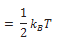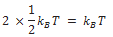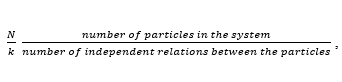The law of equipartition of energy states that if a system is in equilibrium at absolute temperature T, the total energy is distributed equally in different energy modes of absorption, the energy in each mode being equal toEach translational and rotational degree of freedom corresponds to one energy mode of absorption and has energyEach vibration frequency has two modes of energy (kinetic and potential) with corresponding energy equal toUsing the law of equipartition of energy, the molar specific heats of gases can be determined and the values are in agreement with the experimental values of specific heats of several gases. The agreement can be improved by including vibration modes of motion.

## Degrees of Freedom

The degrees of freedom of a dynamical system is defined as the total number of coordinates or independent quantities required to describe completely the position and configuration (arrangement of constituent atoms in space) of the system.

In general, we can say that the number of degree of freedom of a system is equal to the total number of coordinates required to specify the positions of the constituent particles of the particles of the system minus the number of independent relatives existing between the particles.

If ,Then the number of degrees of freedom of the system is f = 3N – k

### Degrees of freedom of a rigid body:

A rigid body of finite size can have both translator and rotator motions.